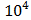# Using a nuclear counter the count rate of emitted particles from a radioactive source is measured. At t=0 it was 1600 counts per second and t=8 seconds it was 100 counts per second. The count rate observed, as counts per second, at t=6 seconds is close to : (a) 150 (b) 360 (c) 200 (d) 400

## Question ID - 50227 :- Using a nuclear counter the count rate of emitted particles from a radioactive source is measured. At t=0 it was 1600 counts per second and t=8 seconds it was 100 counts per second. The count rate observed, as counts per second, at t=6 seconds is close to : (a) 150 (b) 360 (c) 200 (d) 400

3537

at t=0,==1600C/s

at t=8s, A=100C/s=in 8 sec

Therefore half-life is=2 secActivity at t = 6 will be 1600= 200C/scorrect answer is (c).

Next Question :
 If the magnetic field of a plane electromagnetic wave is given by (The speed of light =3×/m/s) B=100×then the maximum electric field associated with it is (a) 4 ×N/C (b) 4.5 ×N/C (c) 6 ×N/C (d) 3 ×N/C